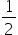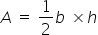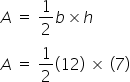Mathematics
Easy

Question

# Area of a triangle A =bh. If b = 12 cm and h = 7 cm, then find the area of the triangle.

## 42 sq.cm    24sq.cm    32sq.cm    None of the aboveHint:

## The correct answer is: 42 sq.cm

### In this question, we are giving the equation for the area of a triangle. The equation consists of two variables, 'b' and ‘h’. The variables are assigned some value. We have to substitute the values and perform the operation.The given expression is.There are two variables in the above expression: ‘l’ and ‘b’. The variables are assigned some value. The value of b=12 cm and the value of h=7cm. And there is one constant.The variables “b” and “h” have a multiplication sign in between them. So the operation we have to perform is multiplication of the variables.After substituting the values, we have to perform the multiplication. To substitute the values, we have to replace “b” by 12 and we have to replace “h” by 7We get the equation as followsA = 42 cm square.Therefore the value of area is 42 cm squared.So option (a), which is ‘42cm’ is the right option.

The main part of such questions is to find the relation between the variables. For that, we have to remember the formulas properly.

### Related Questions to study#### With Turito Foundation.#### Get an Expert Advice From Turito.